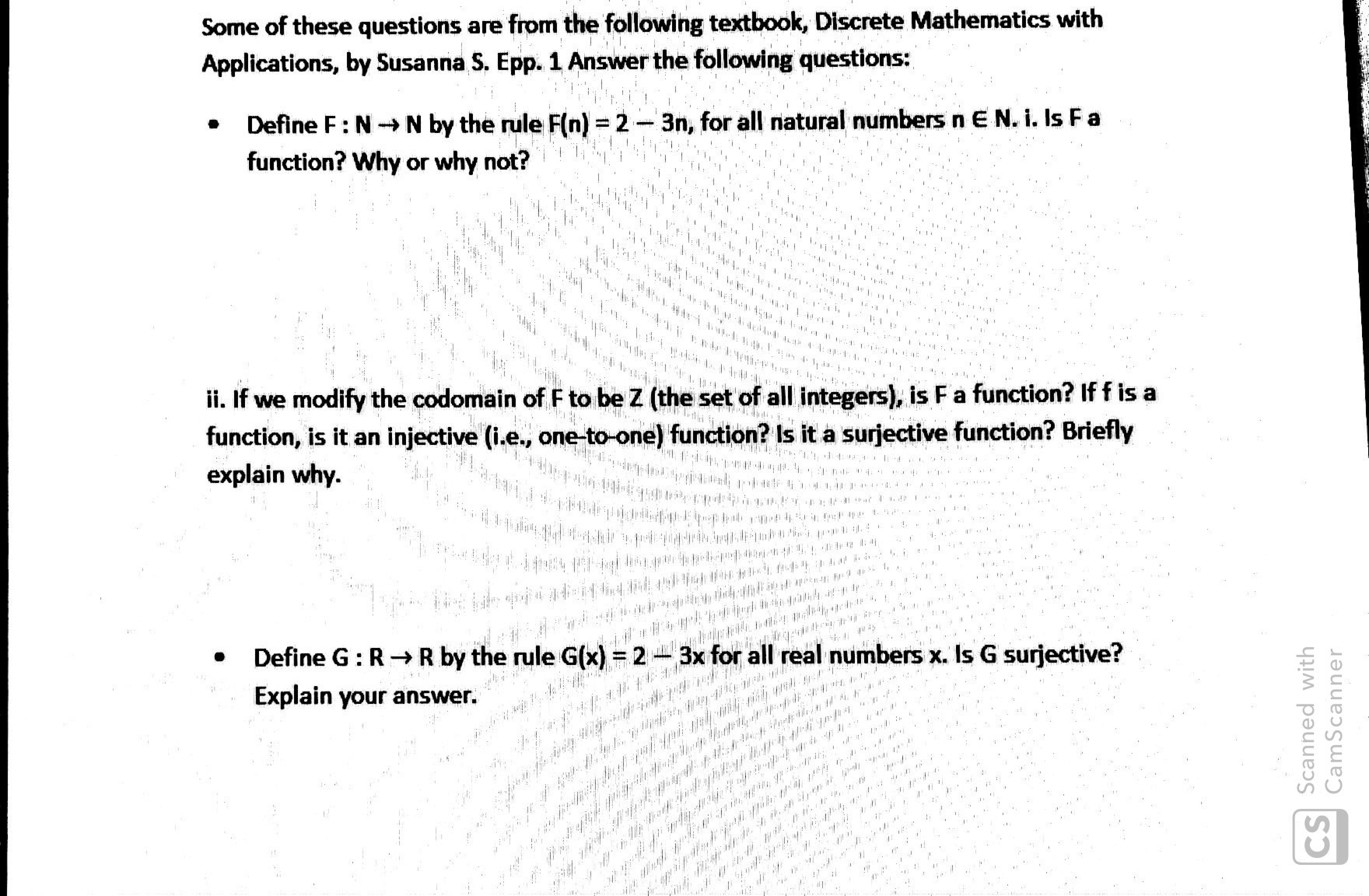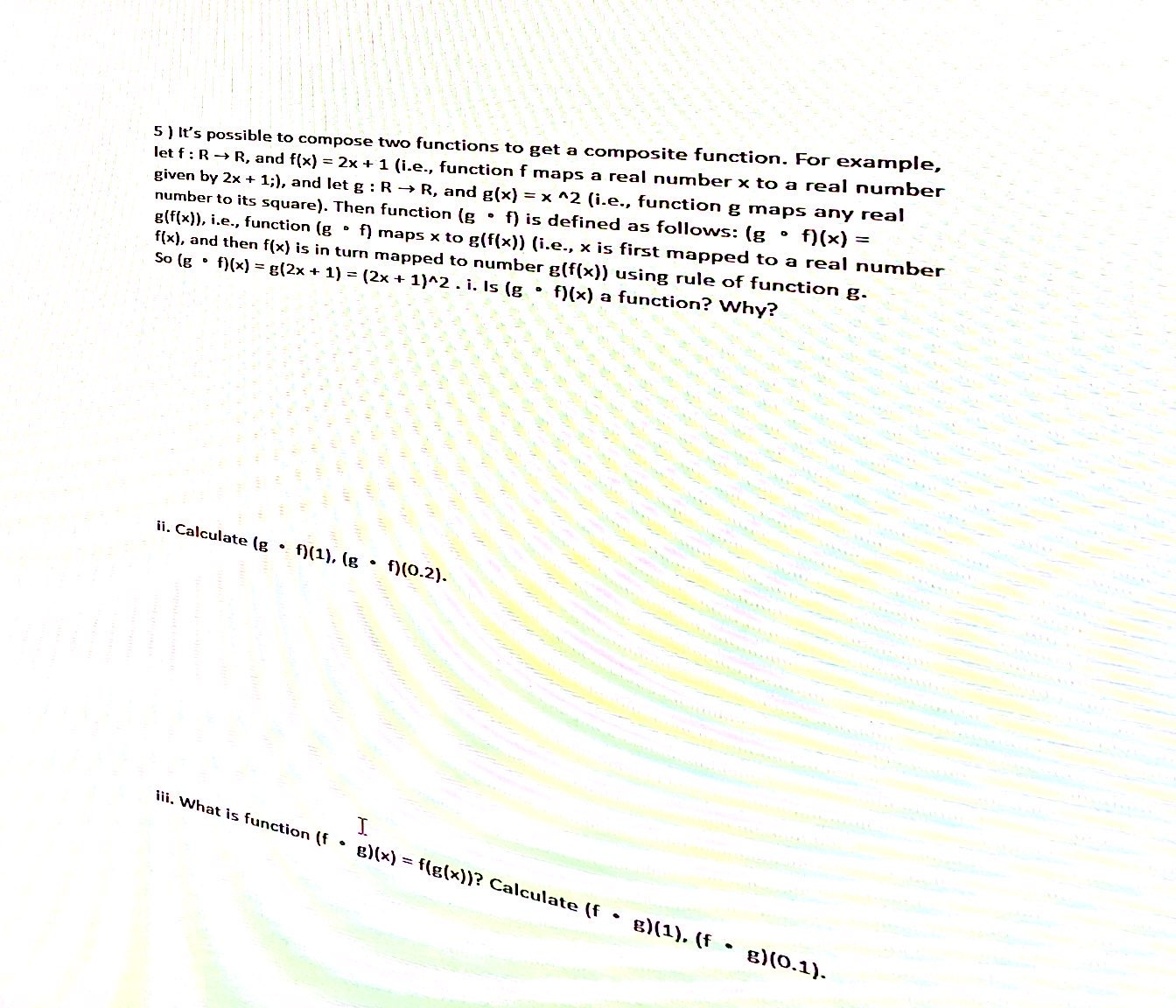# Some of these questions are from the following textbook, Discrete Mathematics with Applications, by Susanna S. Epp. 1 Answer the following questions: Define F: NN by the rule F(n) = 2-3n, for all natural numbers n E N. i. Is Fa function? Why or why not? &ihhe *** 4r152191 i. If we modify the codomain of F to be Z (the set of all integers), is F a function? If f is a function, is it an injective (i.e., one-to-one) function? Is it a surjective function? Briefly explain why. PEWAY I 44**# 4 p Define G: RR by the rule G(x) = 2 3x for all real numbers x. Is G surjective? Explain your answer. /0. a # , CS Scanned with CamScanner 5) It's possible to compose two functions to get a composite function. For example, let f R R, and f(x) 2x 1 (i.e., function f maps a real number x to a real number given by 2x +1;), and let g: RR, and g(x) = x ^2 (i.e., function g maps any real number to its square). Then function (g f) is defined as follows: (g g(f(x)), i.e., function (g f) maps x to g(f(x)) (i.e., x is first mapped to a real number f(x), and then f(x) is in turn mapped to number g(f(x)) using rule of function g. So (g f)(x) g(2x 1) (2x 1)^2.i. Is (g f)(x) a function? Why? f)(x) ii. Calculate (g f)(1), (g. f)(0.2). I ii. What is function (f g)(x)= f(g(x))? Calculate (f g)(1), (f e)(0.1)

Questionhelp_outlineImage TranscriptioncloseSome of these questions are from the following textbook, Discrete Mathematics with Applications, by Susanna S. Epp. 1 Answer the following questions: Define F: NN by the rule F(n) = 2-3n, for all natural numbers n E N. i. Is Fa function? Why or why not? &ihhe *** 4r152191 i. If we modify the codomain of F to be Z (the set of all integers), is F a function? If f is a function, is it an injective (i.e., one-to-one) function? Is it a surjective function? Briefly explain why. PEWAY I 44**# 4 p Define G: RR by the rule G(x) = 2 3x for all real numbers x. Is G surjective? Explain your answer. /0. a # , CS Scanned with CamScanner fullscreenhelp_outlineImage Transcriptionclose5) It's possible to compose two functions to get a composite function. For example, let f R R, and f(x) 2x 1 (i.e., function f maps a real number x to a real number given by 2x +1;), and let g: RR, and g(x) = x ^2 (i.e., function g maps any real number to its square). Then function (g f) is defined as follows: (g g(f(x)), i.e., function (g f) maps x to g(f(x)) (i.e., x is first mapped to a real number f(x), and then f(x) is in turn mapped to number g(f(x)) using rule of function g. So (g f)(x) g(2x 1) (2x 1)^2.i. Is (g f)(x) a function? Why? f)(x) ii. Calculate (g f)(1), (g. f)(0.2). I ii. What is function (f g)(x)= f(g(x))? Calculate (f g)(1), (f e)(0.1) fullscreen

### Want to see the step-by-step answer?